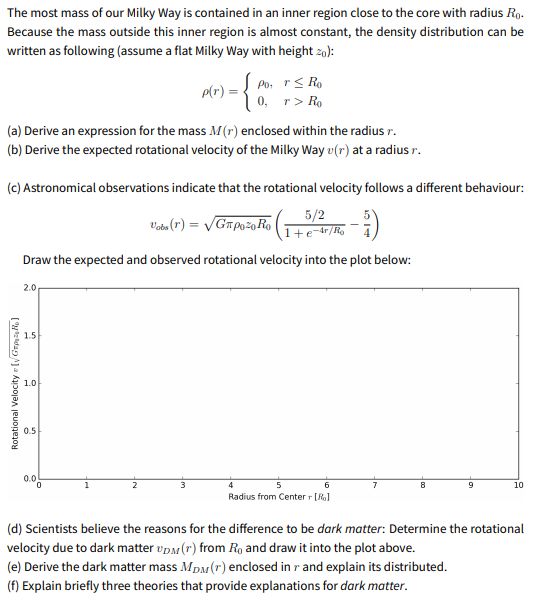# The most mass of our Milky Way is contained in an inner region close to the core with radius Ro. Because the mass outside this inner region is almost constant, the density distribution can be written as following (assume a flat Milky Way with height z0): Po, rs Ro 0, r> Ro p(r) (a) Derive an expression for the mass M(r) enclosed within the radius r. (b) Derive the expected rotational velocity of the Milky Way u(r) at a radius r. (c) Astronomical observations indicate that the rotational velocity follows a different behaviour: Vada (r) = VGapoz0Ro 5/2 1+etr/R ¯ ] Draw the expected and observed rotational velocity into the plot below:

Question

The qs was solved . urgent plzhelp_outlineImage TranscriptioncloseThe most mass of our Milky Way is contained in an inner region close to the core with radius Ro. Because the mass outside this inner region is almost constant, the density distribution can be written as following (assume a flat Milky Way with height z0): Po, rs Ro 0, r> Ro p(r) (a) Derive an expression for the mass M(r) enclosed within the radius r. (b) Derive the expected rotational velocity of the Milky Way u(r) at a radius r. (c) Astronomical observations indicate that the rotational velocity follows a different behaviour: Vada (r) = VGapoz0Ro 5/2 1+etr/R ¯ ] Draw the expected and observed rotational velocity into the plot below: fullscreen

### Want to see this answer and more?

Experts are waiting 24/7 to provide step-by-step solutions in as fast as 30 minutes!*

*Response times may vary by subject and question complexity. Median response time is 34 minutes for paid subscribers and may be longer for promotional offers.
Tagged in
Science